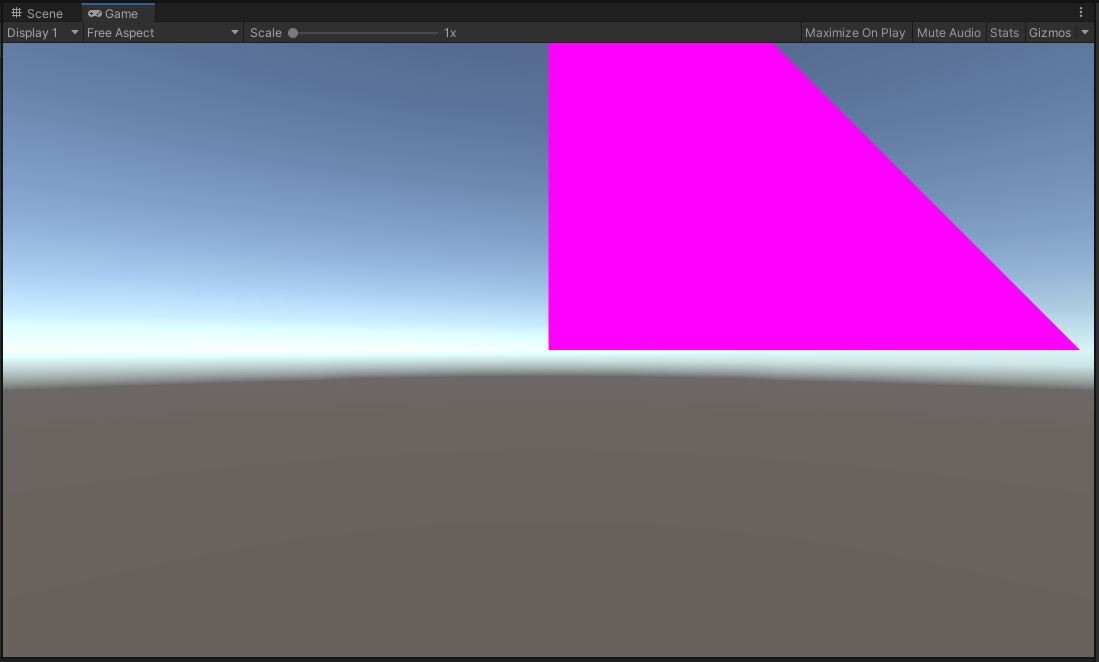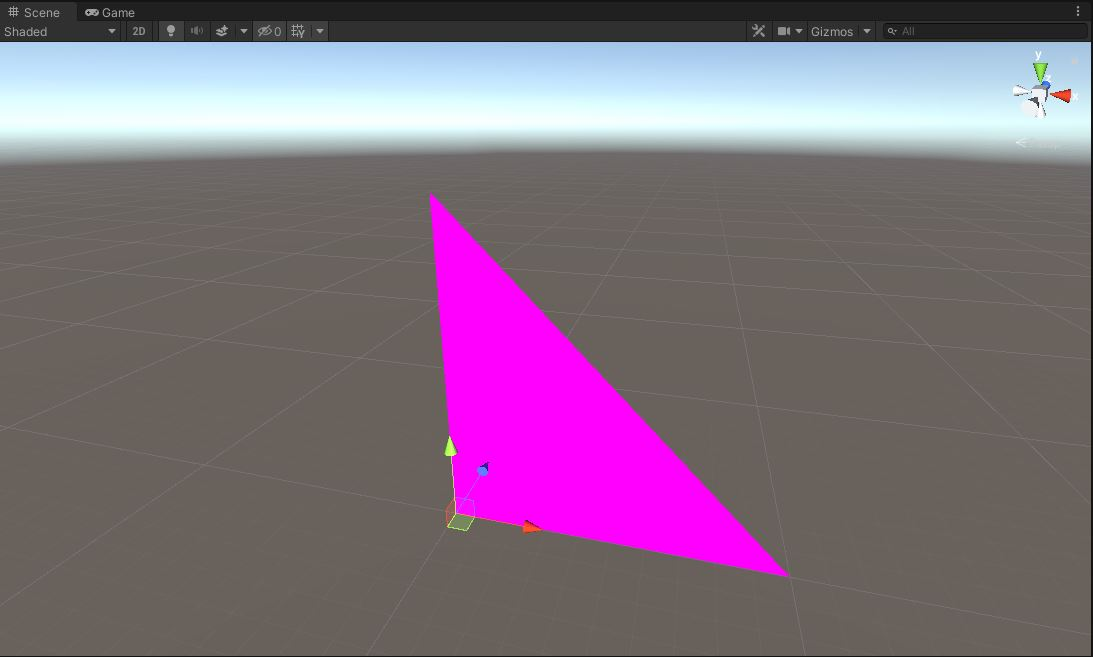# 3. 详论

## 3.1. 准备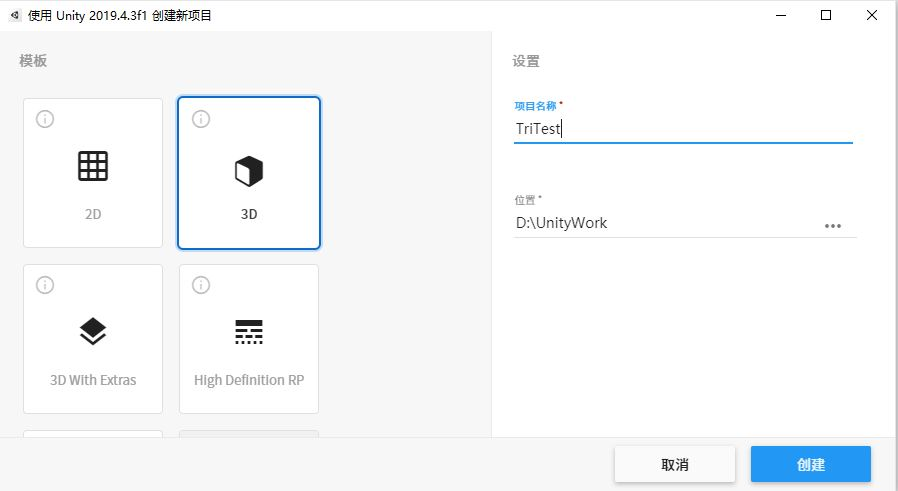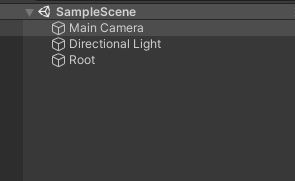GameObject对象是Unity3D中得一个基础类，Unity3D中得绝大部分对象都是基于它实现的，比如相机、灯光、或者模型等。所以我们这里把创建的名为Root的GameObject对象作为场景的根节点。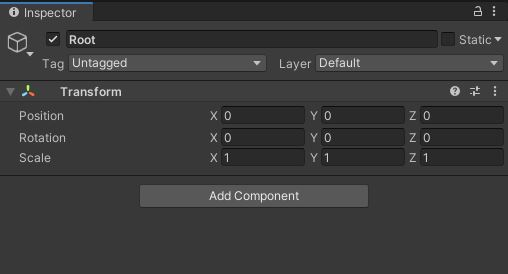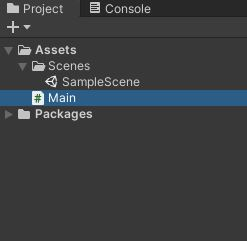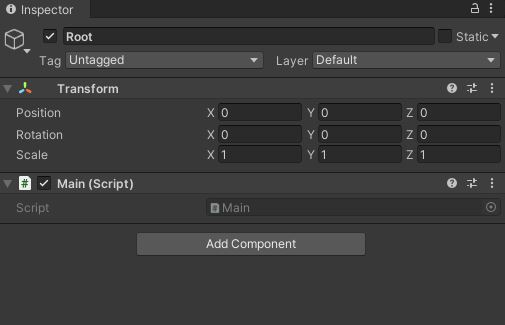## 3.2. 实现

using System.Collections;
using System.Collections.Generic;
using UnityEngine;

public class Main : MonoBehaviour
{
// Start is called before the first frame update
void Start()
{
}

// Update is called once per frame
void Update()
{
}
}


1. Start()表示初始化(第一帧)的时候需要更新的内容，通常用于初始化之后不再更新的内容。比如我们会在这里绘制一个物体。
2. Update()表示每一帧都需要实时更新的内容，比如相机与鼠标键盘事件的交互。

using System.Collections;
using System.Collections.Generic;
using UnityEngine;

public class Main : MonoBehaviour
{
// Start is called before the first frame update
void Start()
{
GameObject main = GameObject.Find("/Root");
if (main == null)
{
return;
}

GameObject triangleGameObject = GreateTriangle();
triangleGameObject.transform.parent = main.transform;
}

GameObject GreateTriangle()
{
string name = "triangle";

Mesh mesh = new Mesh();
mesh.name = name;

Vector3[] vertices = new Vector3
{
new Vector3(0, 0, 0),
new Vector3(0, 10, 0),
new Vector3(10, 0, 0)
};
mesh.vertices = vertices;

int[] triangles = new int { 0, 1, 2 };
mesh.triangles = triangles;

GameObject triangleGameObject = new GameObject(name);
mf.sharedMesh = mesh;

return triangleGameObject;
}

// Update is called once per frame
void Update()
{
}
}


## 3.3. 解析

### 3.3.1. 场景树对象

void Start()
{
GameObject main = GameObject.Find("/Root");
if (main == null)
{
return;
}

GameObject triangleGameObject = GreateTriangle();
triangleGameObject.transform.parent = main.transform;
}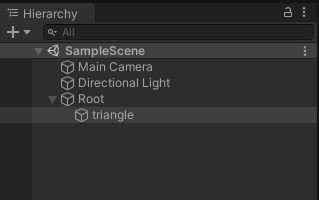### 3.3.2. 绘制方法

GameObject GreateTriangle()
{
string name = "triangle";

Mesh mesh = new Mesh();
mesh.name = name;

Vector3[] vertices = new Vector3
{
new Vector3(0, 0, 0),
new Vector3(0, 10, 0),
new Vector3(10, 0, 0)
};
mesh.vertices = vertices;

int[] triangles = new int { 0, 1, 2 };
mesh.triangles = triangles;

//...
}


GameObject GreateTriangle()
{
//...

GameObject triangleGameObject = new GameObject(name);
mf.sharedMesh = mesh;